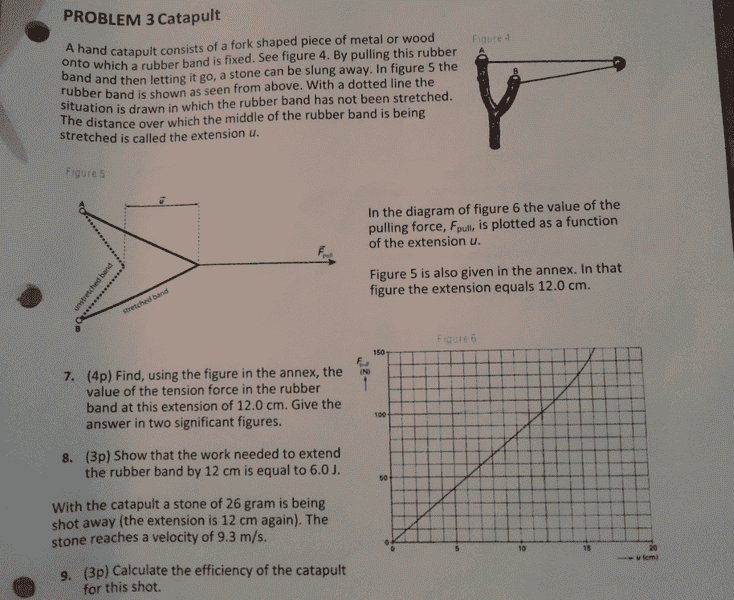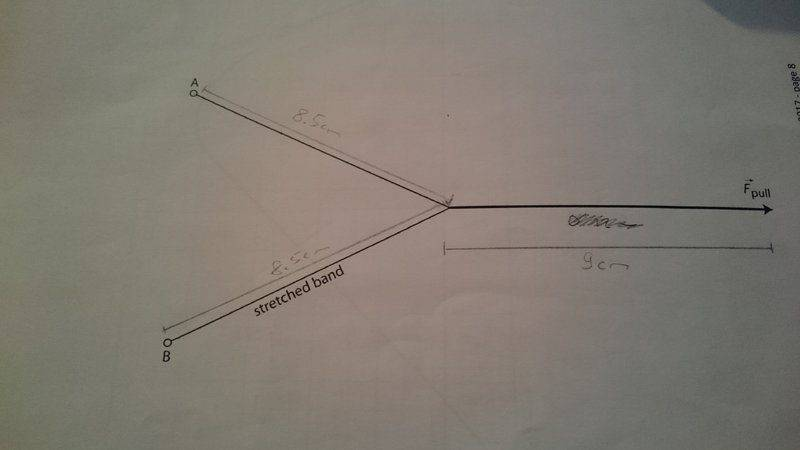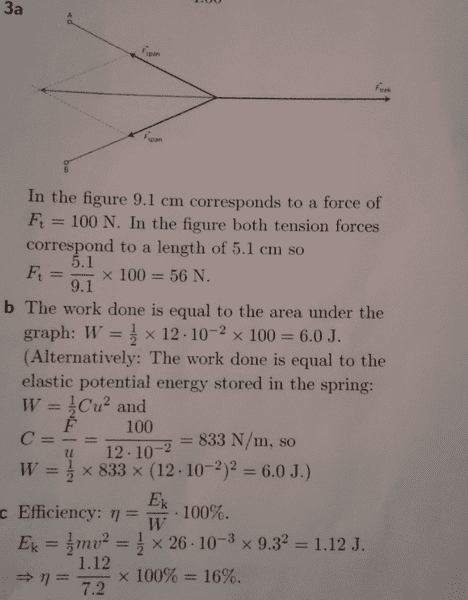Slingshot problem

Homework StatementHomework Equations

Elastic potential energy = 1/2 * C * u^2

Ek = 1/2 * m * v^2

Efficiency = useful output/total input

The Attempt at a Solution

a) My main problem is to find the length of tension force. From the graph i can see that 12 cm corresponds to 100N, so i just need to find the length of the tension in cm and i can determine it in newton. The solution states the tension forces are approximately 5.1cm long but i really cant figure out why, for me it seems they kinda arbitrarily picked a point on the slingshot but this cant be the reason.

b) I got it

c) So if i use the formula for kinetic energy i get: Ek = 1/2 * 26*10^-3 kg * (9.3 ms^-1)^2 = 1.12 J.
But i dont know how to calculate the total energy input for the efficiency in this question. The solution says its 7.2J but i thought it should be the 6.0J from b)

I hope someone can explain it to me, thanks for every answer.

Attachments

BvU
Homework Helper
The meaning of 'extension' of the rubber band is given in the text. Its direction is not along the length of the band but along the direction of the force !
The solution states the tension forces are approximately 5.1cm long
That would really surprise me: tension forces do not have a length.

What surprises me about your picture is that both halves of the rubber band have 8.5 cm. On my screen I measure 9.5 and 10.5 cm, a considerable difference! Did you take the picture at an angle ?
Anyway, the two halves of the band combined exert 100 N. Your task is to decompose that in two tension forces along the two sections of the band. Do you know how to do that ?

I find it confusing that your exercises are numbered 7, 8, 9, and your answers are 'lettered' a), b), c).

Re c) : apparently you have a solution (manual?) at hand. How come it does not help you to undertand part b) ?

I have no idea where the 7.2 J could come from.

i thought i measured correctly but im not sure. The 7,8,9 was a mistake in the problem, they are equivalent to a), b), c). Here are the solutions:But if they have a combined force 100N, then the tension force of one side needs to be 50N, right?

Attachments

BvU
•